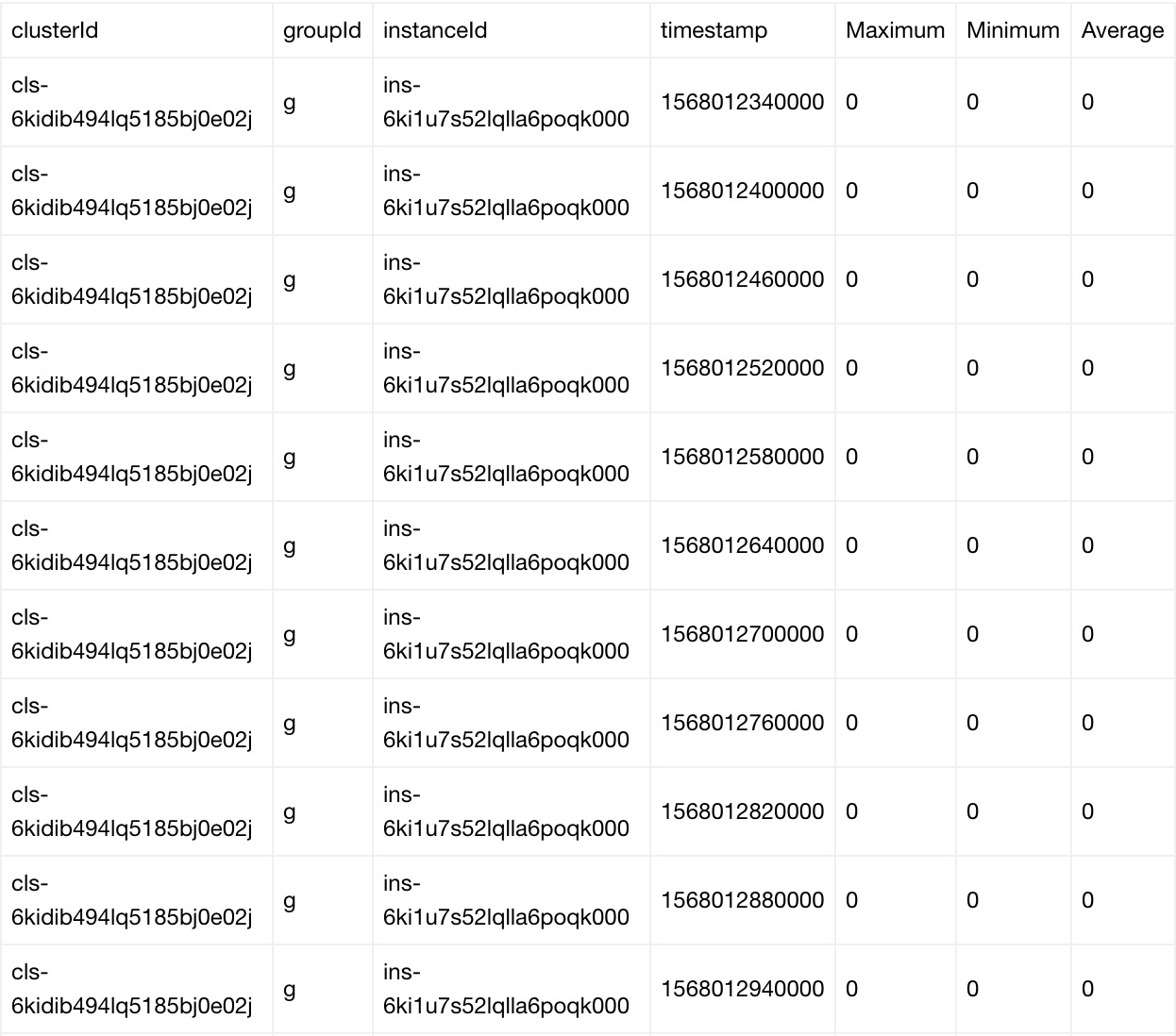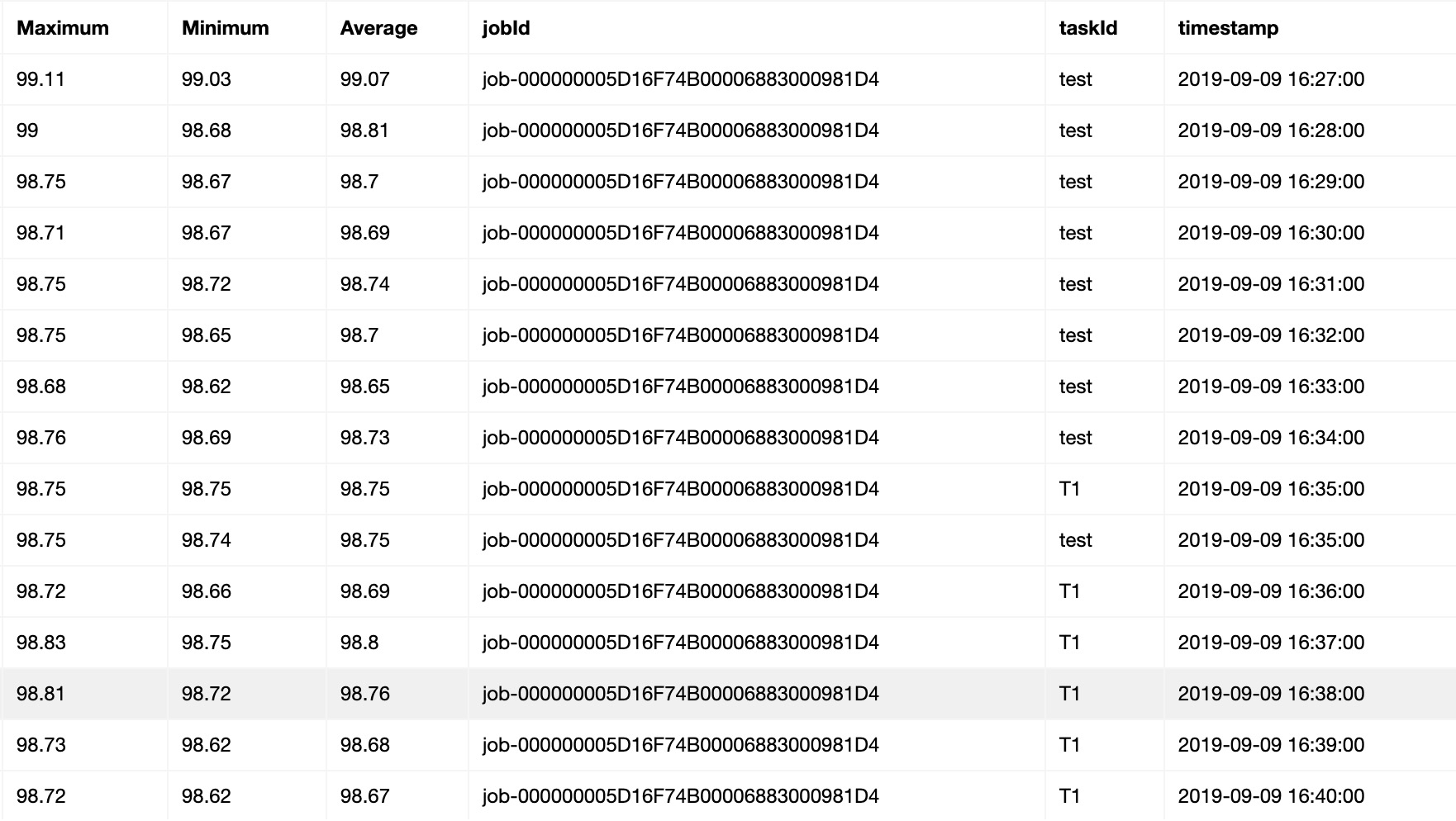Search
Document Center

# Batch Compute:GetBatchMetrics

Last Updated:Jun 19, 2020

This topic describes the metrics used for monitoring resource usage in Batch Compute and how to query the metrics and their statistics. Batch Compute allocates resources for running jobs on clusters. The metrics in Batch Compute are available in the cluster and job dimensions.

# Cluster metrics

## Metrics

The following table describes the metrics that Batch Compute provides in the cluster dimension.

Metric Name Unit Aggregated statistics
cls_dataVfsFsSizePused Data disk usage % Average, maximum, and minimum
cls_systemCpuUtilIdle CPU idle rate % Average, maximum, and minimum
cls_systemCpuUtilUsed CPU usage % Average, maximum, and minimum
cls_vfsFsSizePused System disk usage % Average, maximum, and minimum
cls_vmMemorySizePused Memory usage % Average, maximum, and minimum
• Batch Compute collects the metrics for each instance.
• Batch Compute collects the statistics of each metric by cluster, group, and instance.
• The statistics of each metric include the average, maximum, and minimum values in the past minute.
• By default, Batch Compute pushes the statistics to CloudMonitor every 10 seconds.
• When you call the DescribeMetricData operation to query the statistics of a metric, you can set the Period parameter to specify the period for aggregating the statistics. The default aggregation period is 1 minute.

## Sample statistics# Job metrics

## Metrics

The following table describes the metrics that Batch Compute provides in the job dimension.

Metric Name Unit Aggregated statistics
job_dataVfsFsSizePused Data disk usage % Average, maximum, and minimum
job_systemCpuUtilIdle CPU idle rate % Average, maximum, and minimum
job_systemCpuUtilUsed CPU usage % Average, maximum, and minimum
job_vfsFsSizePused System disk usage % Average, maximum, and minimum
job_vmMemorySizePused Memory usage % Average, maximum, and minimum

## Sample statistics# Use API operations to query metrics and related statistics

Batch Compute pushes the statistics of all metrics to CloudMonitor. You can query the metrics and related statistics of Batch Compute instances by calling the API operations that CloudMonitor provides.

## DescribeMetricMetaList

You can call this operation to query metrics.

## DescribeMetricData

You can call this operation to query the statistics of each metric collected for a cluster or job.

## Sample code

``#!/usr/bin/env python``#coding=utf-8``# https://www.alibabacloud.com/help/doc-detail/51936.htm``import os``import json``import time``import sys``import datetime``from functools import wraps``from aliyunsdkcore.client import AcsClient``from aliyunsdkcore.acs_exception.exceptions import ClientException``from aliyunsdkcore.acs_exception.exceptions import ServerException``from aliyunsdkcms.request.v20190101.DescribeMetricListRequest import DescribeMetricListRequest``from aliyunsdkcms.request.v20190101.DescribeMetricMetaListRequest import DescribeMetricMetaListRequest``akId = 'your key Id'``akKey = 'your key'``region = 'cn-hangzhou'``# jobId = "job-000000005D16F74B00006883000303E9"``jobId = "job-000000005D16F74B00006883000332DD"``def retryWrapper(func):``    @wraps(func)``    def wrapper(*args,**kwargs):``        index = 0``        while True:``            try:``                res = func(*args,**kwargs)``                break``            except Exception,e:``                if index > 6:``                    raise Exception(str(e))``                else:``                    time.sleep(0.5 * pow(2,index))``                    index += 1``        return res``    return wrapper``@retryWrapper``def listBatchMetricMeta(client, objId):``    metrics = []``    request = DescribeMetricMetaListRequest()``    request.set_accept_format('json')``    request.set_Namespace("acs_batchcomputenew")``    response = client.do_action_with_exception(request)``    res = json.loads(response)``    prefix = objId.strip().split("-")``    for metric in res["Resources"]["Resource"]:``        if prefix not in metric["MetricName"]:``            continue``        metrics.append(metric["MetricName"])``    return metrics``@retryWrapper``def getSpecJobMetricsInfo(client, objId, metrics, startTime = None):``    nextToken = None``    request = DescribeMetricListRequest()``    request.set_accept_format('json')``    request.set_Period("60")``    request.set_Length("1000")``    request.set_EndTime(time.strftime("%Y-%m-%d %H:%M:%S", time.localtime(time.time())))``    # By default, the statistics in the past seven days are queried.``    if not startTime:``        sevenDayAgo = (datetime.datetime.now() - datetime.timedelta(days = 7))``        startTime = sevenDayAgo.strftime("%Y-%m-%d %H:%M:%S")``    request.set_StartTime(startTime)``    prefix = objId.strip().split("-")``    if "job" in prefix:``        dimensionInfo = [{"jobId":objId}]``    else:``        dimensionInfo = [{"clusterId":objId}]``    request.set_Dimensions(json.dumps(dimensionInfo))``    request.set_MetricName(metrics)``    request.set_Namespace("acs_batchcomputenew")``    metricsInfo = []``    while True:``        if nextToken:``            request.set_NextToken(nextToken)``        response = client.do_action_with_exception(request)``        res = json.loads(response)``        if res.has_key("Datapoints") and len(res["Datapoints"]):``            metricsInfo.extend(json.loads(res["Datapoints"]))``        else:``            print res``        if res.has_key("NextToken") and res["NextToken"]:``            nextToken = res["NextToken"]``            continue``        else:``            break``    return metricsInfo``if __name__ == "__main__":``    client = AcsClient(akId, akKey, region)``    # metricsName = ['job_systemCpuUtilIdle', 'job_systemCpuLoad', 'job_vmMemorySizePused', 'job_vfsFsSizePused', 'job_dataVfsFsSizePused']``    metricsName = listBatchMetricMeta(client, jobId)``    for metrics in metricsName:``        try:``            ret = getSpecJobMetricsInfo(client, jobId, metrics)``        except Exception,e:``            print "get metrics info failed, %s" % str(e)``            sys.exit(1)``        if not len(ret):``            continue``        # You can aggregate the returned data.``        print ret``
• Before running the sample code, install the Alibaba Cloud SDK for Python by running the following commands:
• pip install aliyun_python_sdk_cms
• pip install aliyun_python_sdk_core
• Make sure that the account with the AccessKey specified in the code has the AliyunCloudMonitorReadOnlyAccess permission. For more information about how to grant permissions, see section 5.2 in Activate Batch Compute.

## Use OpenAPI Explorer to query metrics

Alibaba Cloud provides OpenAPI Explorer to simplify API usage. You can use OpenAPI Explorer to automatically generate the sample code by configuring the basic information required for the target operation.